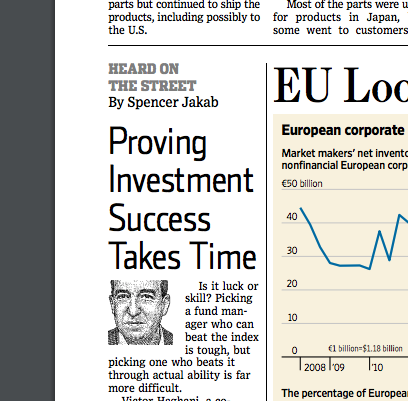Over a million developers have joined DZone.{{announcement.body}}
{{announcement.title}}

# Making Sense of a Probability Problem in the WSJ

DZone 's Guide to

# Making Sense of a Probability Problem in the WSJ

### In this example, are you conducting an experiment with a predetermined sample or are you continuously monitoring the data, stopping when you reach your conclusion?

· Big Data Zone ·
Free Resource

Comment (1)

Save
{{ articles.views | formatCount}} ViewsSomeone wrote to me the other day asking if I could explain a probability example from the Wall Street Journal. ("Proving Investment Success Takes Time," Spencer Jakab, November 25, 2017.)

Victor Haghani ... and two colleagues told several hundred acquaintances who worked in finance that they would flip two coins, one that was normal and the other that was weighted so it came up heads 60% of the time. They asked the people how many flips it would take them to figure out, with a 95% confidence level, which one was the 60% coin. Told to give a "quick guess," nearly a third said fewer than 10 flips, while the median response was 40. The correct answer is 143.

The anecdote is correct in spirit: it takes longer to discover the better of two options than most people suppose. But it's jarring to read that the answer is precisely 143 when the question hasn't been stated clearly.

How many flips would it take to figure out which coin is better with a 95% confidence level? For starters, the answer would have to be a distribution, not a single number. You might quickly come to the right conclusion. You might quickly come to the wrong conclusion. You might flip coins for a long time and never come to a conclusion. Maybe there is a way a formulating the problem so that so that the expected value of the distribution is 143.

How are you to go about flipping the coins? Do you flip both of them, or just flip one coin? For example, you might flip both coins until you are confident that one is better and conclude that the better one is the one that was designed to come up heads 60% of the time. Or you could just flip one of them and test the hypothesis Prob(heads) = 0.5 versus the alternative Prob(heads) = 0.6. Or maybe you flip one coin two times for every one time you flip the other. And so on.

What do you mean by "95% confidence level"? Is this a frequentist confidence interval? And do you compute the (Bayesian) predictive probability of arriving at such a confidence level? Are you computing the (Bayesian) posterior model probabilities of two models, one in which the first coin has a probability of heads 0.5 and the second has a probability 0.6 versus the opposite model?

Do you assume that you know a priori that one coin has a probability of heads 0.5 and the other 0.6, or do you not assume this and just want to find the coin with higher probability of heads, and evaluate such a model when in fact the probabilities of heads are as stated?

Are you conducting an experiment with a predetermined sample size of 143? Or are you continuously monitoring the data, stopping when you reach your conclusion?

I leave it as an exercise to the reader to implement the various alternatives suggested above and see whether one of them produces 143 as a result. (I did a back-of-the-envelope calculation that suggests there is one.) So, the first question is to reverse-engineer which problem statement the article was based on. The second question is to decide which problem formulation you believe would be most appropriate in the context of the article.

Topics:
big data ,probability ,statistics ,data analytics

Comment (1)

Save
{{ articles.views | formatCount}} Views

Published at DZone with permission of

Opinions expressed by DZone contributors are their own.

# {{ parent.title || parent.header.title}}

{{ parent.tldr }}

{{ parent.urlSource.name }}